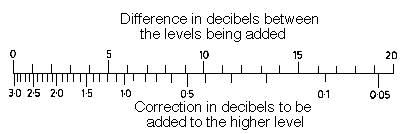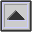SOUND PRESSURE LEVEL (SPL)
 Acoustics / Noise

The term most often used in measuring the magnitude of sound. It is a relative quantity in that it is the ratio between the actual SOUND PRESSURE and a fixed reference pressure. This reference pressure is usually that of the THRESHOLD OF HEARING which has been internationally agreed upon as having the value .0002 dynes/cm2.

SPL may be measured with a SOUND LEVEL METER weighted according to a specific frequency response pattern and termed SOUND LEVEL. The electroacoustic equivalent to SPL is measured with a VU METER.

Because the square of the sound pressure is proportional to SOUND INTENSITY, SPL can be calculated in the same manner and is measured in DECIBELs.

SPL = 10 log (r/rref)2 = 20 log (r/rref)

where r is the given sound pressure and rref is the reference sound pressure.

Two SPL measurements in decibels may be combined with the aid of the following chart. The difference in decibels between the two readings is found on the upper scale, and the corresponding correction appears opposite it on the lower scale. This correction is added to the higher SPL to give the combined measurement. Multiple readings may be combined by repeating this process.

For example, equal SPL readings (0 on top scale) produce a 3.0 increase when combined. A 5 dB difference (say between 60 and 65 dB) produces a 1.2 dB increase (a total of 66.2 dB for the same example). A 10 dB difference requires a 0.4 dB correction, and so on. Also see examples under DECIBEL, INVERSE-SQUARE LAW, Appendix D.Scale for combining sound pressure levels.

home